Next: 6.2.3 Lazy Binomial Queues Up: 6.2 Binomial Queues Previous: 6.2.1 Binomial Queue Operations

## 6.2.2 Binomial Amortized Analysis

Amortized Analysis of Merge

• To merge two binomial queues, an operation similar to addition of binary integers is performed:
At any stage, we may have zero, one, two, or three Bktrees, depending on whether or not the two priority queues contain a Bk tree and whether or not a Bk tree is carried over from the previous step.
• if there is zero or more Bk tree, it is placed as a tree in the resulting binomial queue.
• If there are two, they are merged into a Bk + 1 tree and carried over
• If there are three, one is retained and other two merged.

Result 1:

• A binomial queue of n elements can be built by n successive insertions in 0(n) time.

• Brute force Analysis
Define the cost of each insertions to be
• 1time unit + an extra unit for each linking step
Thus the total will be n units plus the total number of linking steps.
• The 1st, 3rd, ... and each odd-numbered step requires no linking steps since there is no B0 present.
• A quarter of the insertions require only one linking step: 2nd, 6th, 10, ...
• One eighth of insertions require two linking steps.

We could do all this and bound the number of linking steps by n.

The above analysis will not help when we try to analyze a sequence of operations that include more than just insertions.

• Amortized Analysis

Consider the result of an insertion.

• If there is no B0 tree, then the insertion costs one time unit. The result of insertion is that there is now a B0 tree and the forest has one more tree.
• If there is a B0 tree but not B1 tree, then insertion costs 2 time units. The new forest will have a B1 tree but not a B0 tree. Thus number of trees in the forest is unchanged.
• An insertion that costs 3 time units will create a B2 tree but destroy a B0 and B1, yielding one less tree in the forest.
• In general, an insertion that costs c units results in a net increase of 2 - c trees. Since
• a Bc - 1 tree is created
• all Bi trees, 0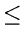ic - 1 are removed.
Thus expensive insertions remove trees and cheap insertions create trees.

 Let ti =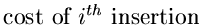ci =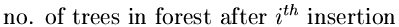We have

 c0 = 0 ti + (ci - ci - 1) = 2

Result 2:

• The amortized running times of Insert, Delete-min, and Merge are 0(1), 0(log n), and 0(log n) respectively.
Potential function = # of trees in the queue
To prove this result we choose:

• Insertion

 ti =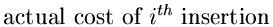ci =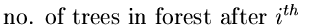ai = ti + (ci - ci - 1) ai = 2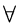i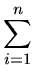ti =ai - (cn - c0) = 2n - (cn - c0)

As long as (cn - c0) is positive, we are done.

In any case (cn - c0) is bounded by log n if we start with an empty tree.

• Merge:

Assume that the two queues to be merged have n1 and n2nodes with T1 and T2 trees. Let n = n1+ n2. Actual time to perform merge is given by:

 ti = 0(logn1 + logn2) = 0(max(logn1, logn2) = 0(log n)

(ci - ci - 1) is at most (log n) since there can be at most (log n) trees after merge.

• Deletemin:
The analysis here follows the same argument as for merge.Next: 6.2.3 Lazy Binomial Queues Up: 6.2 Binomial Queues Previous: 6.2.1 Binomial Queue Operations
eEL,CSA_Dept,IISc,Bangalore